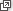## Content

### Non-exponential growth and decay

All the quantities we have seen so far, such as populations and radioactive samples, grow or decay exponentially. That is, they are exponential functions of time $$t$$. However, many quantities in the world grow or decay in a non-exponential fashion.

For instance, the electric field strength $$E$$ of a charged particle decreases rapidly as you move away from the particle. At a distance of $$r$$ from the particle, the field's strength is

$E = \dfrac{C}{r^2},$

where $$C$$ is a constant. The electric field strength is said to obey an inverse-square law, rather than an exponential law; the 'decay' involves $$r^{-2}$$ rather than $$e^{-r}$$.

Many other quantities in the world — such as gravitational force, sound intensity and radio signal strength — obey inverse-square laws.

Another interesting example is Zipf's law, which concerns spoken language. Suppose we rank all the words in a language according to their frequency as spoken. Zipf's law states that the frequency $$f$$ in spoken language of the word ranked $$r$$ is inversely proportional to $$r$$. That is,

$f = \dfrac{C}{r},$

for some constant $$C$$. In other words, word frequency decreases in inverse proportion to rank. (See Wikipediafor more on Zipf's law.)

In addition to these examples, many quantities grow non-exponentially. For instance, if you travel in a straight line at constant velocity $$v$$, then your displacement $$s$$ grows linearly with time, $$s = vt$$. If you travel in a straight line, initially stationary, with constant acceleration $$a$$, then your velocity $$v$$ grows linearly with time, $$v=at$$, and your displacement grows quadratically with time, $$s = \dfrac{1}{2} at^2$$. See the module Motion in a straight line for further details.

When two quantities $$x$$ and $$y$$ are related by an equality of the form $$y = C x^\alpha$$, where $$C$$ and $$\alpha$$ are non-zero constants, we say they obey a power-law relationship. The examples from this section are all power-law relationships:

• inverse-square decay ($$\alpha = -2$$)
• Zipf's law ($$\alpha = -1$$)
• linear growth ($$\alpha = 1$$)
• quadratic growth ($$\alpha = 2$$).

Exponential growth or decay is not a power-law relationship.

We will consider power-law relationships further in the section History and applications (Logarithmic plots).

Next page - Links forward - Discrete and continuous growth